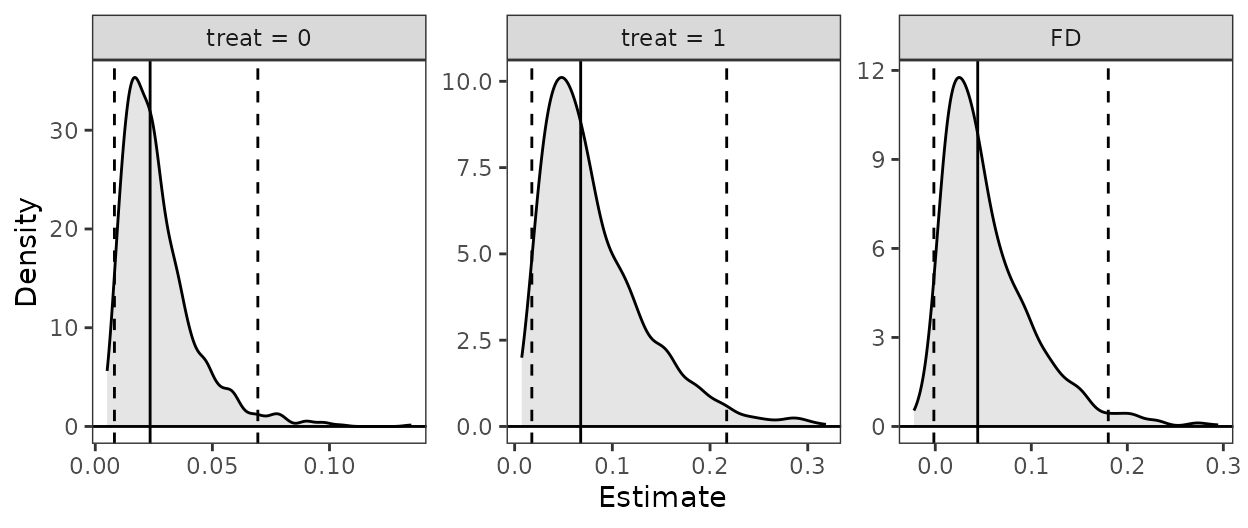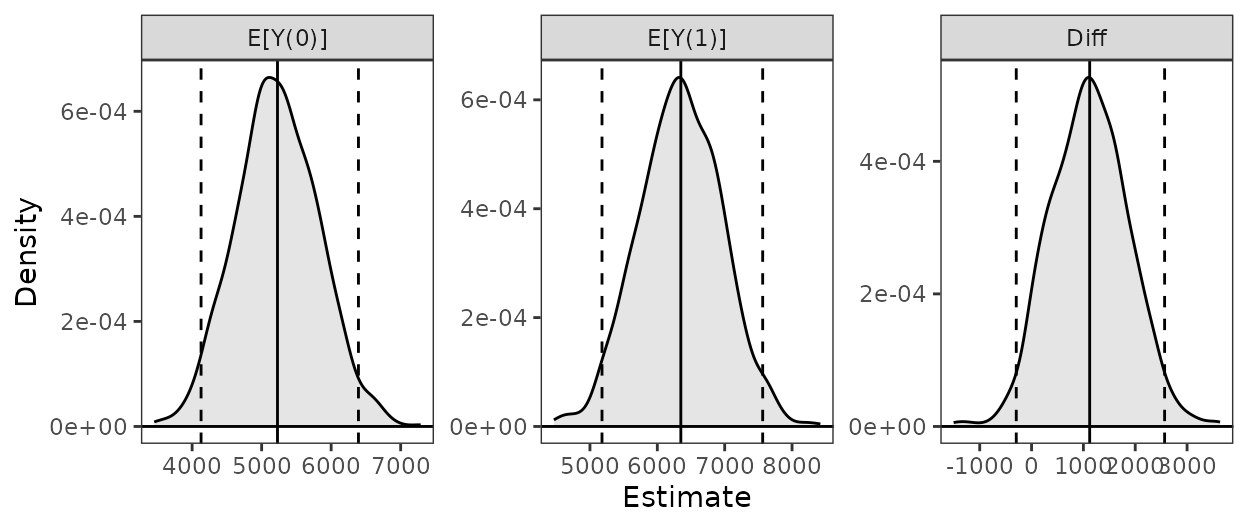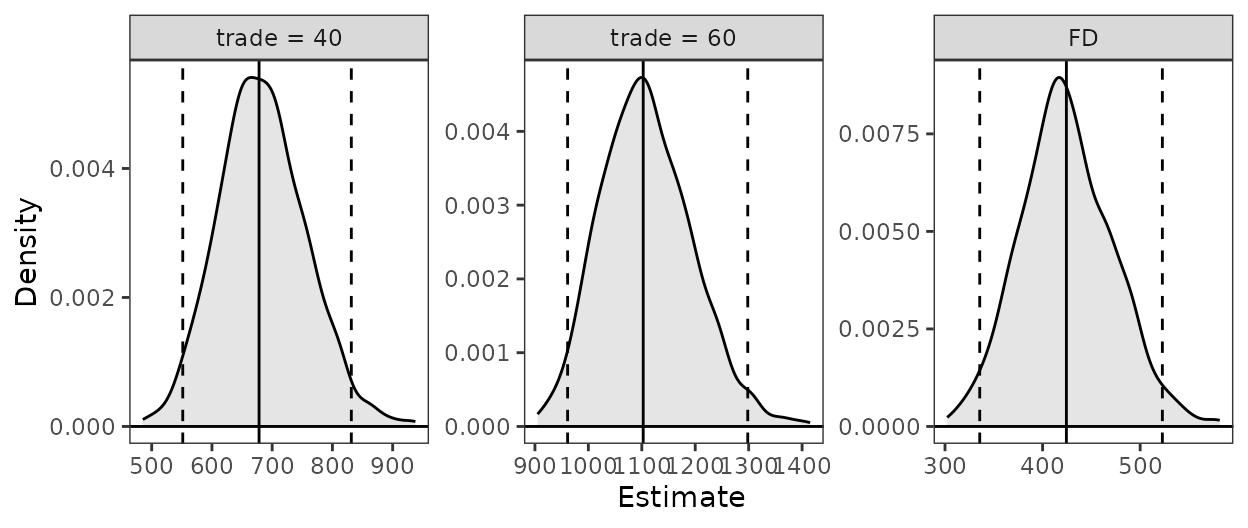## Introduction

In this document, we demonstrate some common uses of Zelig (Imai, King, and Lau 2008) and how the same tasks can be performed using clarify. We’ll include examples for computing predictions at representative values (i.e., setx() and sim() in Zelig), the rare-events logit model, estimating the average treatment effect (ATT) after matching, and combining estimates after multiple imputation.

The usual workflow in Zelig is to fit a model using zelig(), specify quantities of interest to simulate using setx() on the zelig() output, and then simulate those quantities using sim(). clarify uses a similar approach, except that the model is fit outside clarify using functions in a different R package. In addition, clarify’s sim_apply() allows for the computation of any arbitrary quantity of interest. We’ll demonstrate how to replicate a standard Zelig analysis using clarify step-by-step. Because simulation-based inference involves randomness and some of the algorithms may not perfectly align, one shouldn’t expect results to be identical, though in most cases, they should be similar.

## library("Zelig")
library("clarify")
set.seed(100)

Note that both Zelig and clarify have a function called “sim()”, so we will always make it clear which package’s sim() is being used.

## Predictions at representative values

Here we’ll use the lalonde dataset in MatchIt and fit a linear model for re78 as a function of the treatment treat and covariates.

data("lalonde", package = "MatchIt")

We’ll be interested in the predicted values of the outcome for a typical unit at each level of treatment and their first difference.

### Zelig workflow

In Zelig, we fit the model using zelig():

fit <- zelig(re78 ~ treat + age + educ + married + race +
nodegree + re74 + re75, data = lalonde,
model = "ls", cite = FALSE)

Next, we use setx() and setx1() to set our values of treat:

fit <- setx(fit, treat = 0)
fit <- setx1(fit, treat = 1)

Next we simulate the values using sim():

fit <- Zelig::sim(fit)

Finally, we can print and plot the predicted values and first differences:

fit
plot(fit)

### clarify workflow

In clarify, we fit the model using functions outside clarify, like stats::lm()or fixest::feols().

fit <- lm(re78 ~ treat + age + educ + married + race +
nodegree + re74 + re75, data = lalonde)

Next, we simulate the model coefficients using clarify::sim():

s <- clarify::sim(fit)

Next, we use sim_setx() to set our values of the predictors:

est <- sim_setx(s, x = list(treat = 0), x1 = list(treat = 1),
verbose = FALSE)

Finally, we can summarize and plot the predicted values:

summary(est)
#>           Estimate   2.5 %  97.5 %
#> treat = 0   6686.0  5305.0  7986.6
#> treat = 1   8234.3  6416.7 10025.4
#> FD          1548.2   -18.9  3056.8

plot(est)## Rare-events logit

Zelig uses a special method for logistic regression with rare events as described in King and Zeng (2001). This is the primary implementation of the method in R. However, newer methods have been developed that perform similarly to or better than the method of King and Zeng (Puhr et al. 2017) and are implemented in R packages that are compatible with clarify, such as logistf and brglm2.

Here, we’ll use the lalonde dataset with a constructed rare outcome variable to demonstrate how to perform a rare events logistic regression in Zelig and in clarify.

data("lalonde", package = "MatchIt")

#Rare outcome: 1978 earnings over $20k; ~6% prevalence lalonde$re78_20k <- lalonde\$re78 >= 20000

### Zelig workflow

In Zelig, we fit a rare events logistic model using zelig() with model = "relogit".

fit <- zelig(re78_20k ~ treat + age + educ + married + race +
nodegree + re74 + re75, data = lalonde,
model = "relogit", cite = FALSE)

fit

We can compute predicted values at representative values using setx() and Zelig::sim() as above.

fit <- setx(fit, treat = 0)
fit <- setx1(fit, treat = 1)

fit <- Zelig::sim(fit)

fit
plot(fit)

### clarify workflow

Here, we’ll use logistf::logistif() with flic = TRUE, which performs a variation on Firth’s logistic regression with a correction for bias in the intercept (Puhr et al. 2017).

fit <- logistf::logistf(re78_20k ~ treat + age + educ + married + race +
nodegree + re74 + re75, data = lalonde,
flic = TRUE)

summary(fit)
#> logistf::logistf(formula = re78_20k ~ treat + age + educ + married +
#>     race + nodegree + re74 + re75, data = lalonde, flic = TRUE)
#>
#> Model fitted by Penalized ML
#> Coefficients:
#>                      coef     se(coef)    lower 0.95    upper 0.95      Chisq
#> (Intercept) -9.313465e+00 2.036981e+00 -1.330595e+01 -5.3209820512 24.4427501
#> treat        1.106769e+00 6.165332e-01 -1.029333e-01  2.3139987641  3.2185792
#> age          4.144350e-02 2.147498e-02 -8.432745e-04  0.0825427498  3.6937075
#> educ         2.936673e-01 1.283624e-01  4.612509e-02  0.5477908006  5.4633187
#> married      2.909164e-01 4.769332e-01 -6.309243e-01  1.2240602694  0.3844676
#> racehispan   1.056388e+00 7.276772e-01 -4.150796e-01  2.4305568008  2.0440063
#> racewhite    5.431919e-01 6.160352e-01 -6.255328e-01  1.7838676828  0.8008622
#> nodegree     2.868319e-01 5.852738e-01 -8.531608e-01  1.4243907533  0.2462839
#> re74         1.108995e-04 2.821452e-05  5.775266e-05  0.0001664918 17.0427331
#> re75         4.457916e-05 4.720736e-05 -4.706292e-05  0.0001334568  0.9472092
#>                        p method
#> (Intercept) 7.655103e-07      1
#> treat       7.280680e-02      2
#> age         5.461808e-02      2
#> educ        1.941973e-02      2
#> married     5.352219e-01      2
#> racehispan  1.528067e-01      2
#> racewhite   3.708357e-01      2
#> nodegree    6.197040e-01      2
#> re74        3.654797e-05      2
#> re75        3.304307e-01      2
#>
#> Method: 1-Wald, 2-Profile penalized log-likelihood, 3-None
#>
#> Likelihood ratio test=65.80085 on 9 df, p=1.007627e-10, n=614
#> Wald test = 179.4243 on 9 df, p = 0

We can compute predictions at representative values using clarify::sim() and sim_setx().

s <- clarify::sim(fit)

est <- sim_setx(s, x = list(treat = 0), x1 = list(treat = 1),
verbose = FALSE)

summary(est)
#>           Estimate    2.5 %   97.5 %
#> treat = 0  0.02341  0.00891  0.06213
#> treat = 1  0.06760  0.01972  0.22091
#> FD         0.04419 -0.00318  0.19222
plot(est)## Estimating the ATT after matching

Here we’ll use the lalonde dataset and perform propensity score matching and then fit a linear model for re78 as a function of the treatment treat, the covariates, and their interaction. From this model, we’ll compute the ATT of treat using Zelig and clarify.

data("lalonde", package = "MatchIt")

m.out <- MatchIt::matchit(treat ~ age + educ + married + race +
nodegree + re74 + re75, data = lalonde,
method = "nearest")

### Zelig workflow

In Zelig, we fit the model using zelig() directly on the matchit object:

fit <- zelig(re78 ~ treat * (age + educ + married + race +
nodegree + re74 + re75),
data = m.out, model = "ls", cite = FALSE)

Next, we use ATT() to request the ATT of treat and simulate the values:

fit <- ATT(fit, "treat")
fit
plot(fit)

### clarify workflow

In clarify, we need to extract the matched dataset and fit a model outside clarify using another package.

m.data <- MatchIt::match.data(m.out)

fit <- lm(re78 ~ treat * (age + educ + married + race +
nodegree + re74 + re75),
data = m.data)

Next, we simulate the model coefficients using clarify::sim(). Because we performed pair matching, we will request a cluster-robust standard error:

s <- clarify::sim(fit, vcov = ~subclass)

Next, we use sim_ame() to request the average marginal effect of treat within the subset of treated units:

est <- sim_ame(s, var = "treat", subset = treat == 1,
contrast = "diff", verbose = FALSE)

Finally, we can summarize and plot the ATT:

summary(est)
#>         Estimate 2.5 % 97.5 %
#> E[Y(0)]     5228  4060   6355
#> E[Y(1)]     6349  5226   7468
#> Diff        1121  -474   2635

plot(est)## Combining results after multiple imputation

Here we’ll use the africa dataset in Amelia to demonstrate combining estimates after multiple imputation. This analysis is also demonstrated using clarify at the end of vignette("clarify").

library(Amelia)
data("africa", package = "Amelia")

First we multiply impute the data using amelia() using the specification in the Amelia documentation.

# Multiple imputation
a.out <- amelia(x = africa, m = 10, cs = "country",
ts = "year", logs = "gdp_pc", p2s = 0)

### Zelig workflow

With Zelig, we can supply the amelia object directly to the data argument of zelig() to fit a model in each imputed dataset:

fit <- zelig(gdp_pc ~ infl * trade, data = a.out,
model = "ls", cite = FALSE)

Summarizing the coefficient estimates after the simulation can be done using summary():

summary(fit)

We can use Zelig::sim() and setx() to compute predictions at specified values of the predictors:

fit <- setx(fit, infl = 0, trade = 40)
fit <- setx1(fit, infl = 0, trade = 60)

fit <- Zelig::sim(fit)

Zelig does not allow you to combine predicted values across imputations.

fit
plot(fit)

### clarify workflow

clarify does not combine coefficients, unlike zelig(); instead, the models should be fit using Amelia::with(). To view the combined coefficient estimates, use Amelia::mi.combine().

#Use Amelia functions to model and combine coefficients
fits <- with(a.out, lm(gdp_pc ~ infl * trade))

mi.combine(fits)
#> # A tibble: 4 × 8
#>   term        estimate std.error statistic  p.value       df       r miss.info
#>   <chr>          <dbl>     <dbl>     <dbl>    <dbl>    <dbl>   <dbl>     <dbl>
#> 1 (Intercept) -177.      117.        -1.51 1.87e+ 0  130515. 0.00837   0.00832
#> 2 infl          12.3       9.36       1.31 1.91e- 1 1210309. 0.00273   0.00273
#> 3 trade         21.3       1.82      11.7  1.26e-31   83428. 0.0105    0.0104
#> 4 infl:trade    -0.290     0.140     -2.07 1.96e+ 0  954984. 0.00308   0.00307

Derived quantities can be computed using clarify::misim() and sim_apply() or its wrappers on the with() output, which is a list of regression model fits:

#Simulate coefficients, 100 in each of 10 imputations
s <- misim(fits, n = 100)

#Compute predictions at specified values
est <- sim_setx(s, x = list(infl = 0, trade = 40),
x1 = list(infl = 0, trade = 60),
verbose = FALSE)

summary(est)
#>            Estimate 2.5 % 97.5 %
#> trade = 40      675   570    783
#> trade = 60     1101  1024   1174
#> FD              426   353    498

plot(est)Imai, Kosuke, Gary King, and Olivia Lau. 2008. “Toward a Common Framework for Statistical Analysis and Development.” Journal of Computational and Graphical Statistics 17 (4): 892–913. https://doi.org/10.1198/106186008X384898.
King, Gary, and Langche Zeng. 2001. “Logistic Regression in Rare Events Data.” Political Analysis 9 (2): 137–63. https://doi.org/10.1093/oxfordjournals.pan.a004868.
Puhr, Rainer, Georg Heinze, Mariana Nold, Lara Lusa, and Angelika Geroldinger. 2017. “Firth’s Logistic Regression with Rare Events: Accurate Effect Estimates and Predictions?” Statistics in Medicine 36 (14): 2302–17. https://doi.org/10.1002/sim.7273.